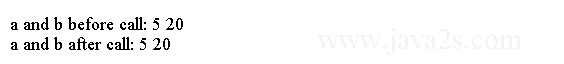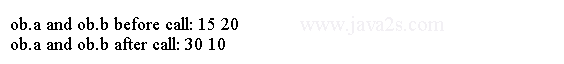# Java Tutorial - Java Variable Type

## Java Object Reference Variable

Object reference variables act differently when an assignment takes place.

For example,

```Box b1 = new Box();
Box b2 = b1;
```

After this fragment executes, `b1` and `b2` will both refer to the same object.

The assignment of b1 to b2 did not allocate any memory or copy any part of the original object. It simply makes b2 refer to the same object as does b1. Thus, any changes made to the object through b2 will affect the object to which b1 is referring.

A subsequent assignment to b1 will simply unhook b1 from the original object without affecting the object or affecting b2.

For example:

```Box b1 = new Box();
Box b2 = b1;
// ...
b1 = null;
```

Here, b1 has been set to null, but b2 still points to the original object.

Java Object Reference Variable

```
class Box {//from  ww  w  . jav  a2 s .c  o  m
int width;
int height;
int depth;
}

public class Main {
public static void main(String args[]) {
Box myBox1 = new Box();
Box myBox2 = myBox1;

myBox1.width = 10;

myBox2.width = 20;

System.out.println("myBox1.width:" + myBox1.width);
System.out.println("myBox2.width:" + myBox2.width);
}
}
```

The code above generates the following result.## Java Method Argument Passing

When a parameter is passed into a method, it can be passed by value or by reference. Pass-by-value copies the value of an argument into the parameter. Changes made to the parameter have no effect on the argument. Pass-by-reference passes a reference to the parameter. Changes made to the parameter will affect the argument.

When a simple primitive type is passed to a method, it is done by use of call-by-value. Objects are passed by use of call-by-reference.

The following program uses the "pass by value".

```
class Test {//from  w ww  . j  a  v a  2s.co  m
void change(int i, int j) {
i *= 2;
j /= 2;
}
}
public class Main {
public static void main(String args[]) {
Test ob = new Test();

int a = 5, b = 20;

System.out.println("a and b before call: " + a + " " + b);

ob.change(a, b);

System.out.println("a and b after call: " + a + " " + b);
}
}
```

The output from this program is shown here:## Example

In the following program, objects are passed by reference.

```
class Test {//  ww  w . j  av  a 2s.  c  om
int a, b;
Test(int i, int j) {
a = i;
b = j;
}
void meth(Test o) {
o.a *= 2;
o.b /= 2;
}
}
public class Main {
public static void main(String args[]) {
Test ob = new Test(15, 20);

System.out.println("ob.a and ob.b before call: " + ob.a + " " + ob.b);

ob.meth(ob);

System.out.println("ob.a and ob.b after call: " + ob.a + " " + ob.b);
}
}
```

This program generates the following output: